Courses

# Systems Of Linear Equations, Matrix Algebra And Transform Theory - MCQ Test 2

## 30 Questions MCQ Test Topicwise Question Bank for GATE Electrical Engineering | Systems Of Linear Equations, Matrix Algebra And Transform Theory - MCQ Test 2

Description
This mock test of Systems Of Linear Equations, Matrix Algebra And Transform Theory - MCQ Test 2 for Civil Engineering (CE) helps you for every Civil Engineering (CE) entrance exam. This contains 30 Multiple Choice Questions for Civil Engineering (CE) Systems Of Linear Equations, Matrix Algebra And Transform Theory - MCQ Test 2 (mcq) to study with solutions a complete question bank. The solved questions answers in this Systems Of Linear Equations, Matrix Algebra And Transform Theory - MCQ Test 2 quiz give you a good mix of easy questions and tough questions. Civil Engineering (CE) students definitely take this Systems Of Linear Equations, Matrix Algebra And Transform Theory - MCQ Test 2 exercise for a better result in the exam. You can find other Systems Of Linear Equations, Matrix Algebra And Transform Theory - MCQ Test 2 extra questions, long questions & short questions for Civil Engineering (CE) on EduRev as well by searching above.
QUESTION: 1

### Consider the system of equations given below:  x + y =  2 2x + 2y = 5 This system has

Solution:

(b) This can be written as AX = B Where A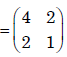Angemented matrix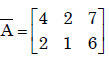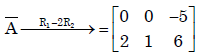rank(A) ≠ rank(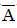). The system is inconsistant .So system has no solution.

QUESTION: 2

### For what value of a, if any, will the following system of equations in x, y and z have a solution?   2x + 3y = 4 x+y+z = 4 x + 2y - z = a

Solution:

(b)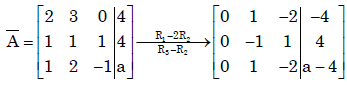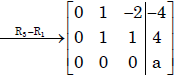If a = 0 then rank (A) = rank() = 2. Therefore the system is consistant
∴ The system has soln .

QUESTION: 3

### Solution for the system defined by the set of equations 4y + 3z = 8; 2x – z = 2 and 3x + 2y =5 is

Solution:

Ans.(d)
Consider the matrix A =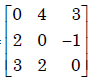,Now det (A) = 0
So byCramer's Rule the system has no solution

QUESTION: 4

For what values of α and β the following simultaneous equations have an infinite numberof solutions?
x + y + z = 5; x + 3y + 3z = 9; x + 2y + αz = β

Solution:

(d)=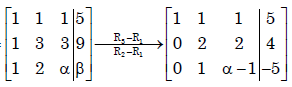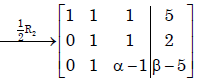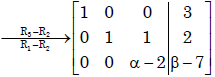For infinite solution of the system
α − 2 = 0 and β − 7 = 0
⇒ α = 2 and β = 7.

QUESTION: 5

Let A be a 3 × 3 matrix with rank 2. Then AX = 0 has

Solution:

(b)
We know , rank (A) + Solution space X(A) = no. of unknowns.
⇒2 + X(A) = 3 . [Solution space X(A)= No. of linearly independent vectors]
⇒ X(A) =1.

QUESTION: 6

A is a 3 x 4 real matrix and A x = b is an inconsistent system of equations. The highest possible rank of A is

Solution:

(b). Highest possible rank of A= 2 ,as Ax = b is an inconsistent system.

QUESTION: 7

Consider the matrices X (4 × 3), Y (4 × 3) and P (2 × 3). The order or P (XTY)–1PT] T will be

Solution:

(a)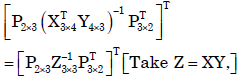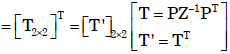QUESTION: 8

Given matrix [A] =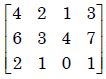the rank of the matrix is

Solution:

(c)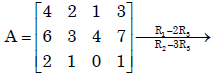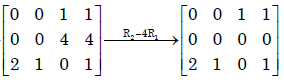∴Rank(A) = 2

QUESTION: 9

The Laplace transform of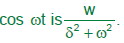Solution:

Ans. (b)False
Laplace transform of

QUESTION: 10

There are two containers, with one containing 4 Red and 3 Green balls and the other containing Blue and 4 Green balls. One bal is drawn at random form each container.The probability that one of the ball is Red and the other is Blue will be

Solution:
QUESTION: 11

If L defines the Laplace Transform of a function, L [sin (at)] will be equal to

Solution:

Ans. (b)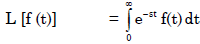⇒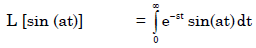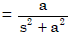QUESTION: 12

The Inverse Laplace transform of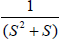is

Solution:

Ans. (c)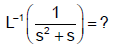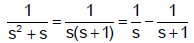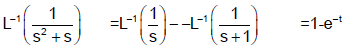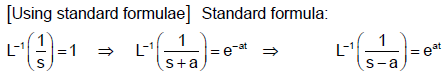QUESTION: 13

Laplace transform for the function f(x) = cosh (ax) is

Solution:

Ans. (b)
It is a standard result that
L (cosh at) =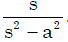QUESTION: 14

If F(s) is the Laplace transform of function f (t), then Laplace transform of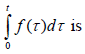Solution:

Ans. (a)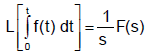QUESTION: 15

Laplace transform of the function sin ωt

Solution:

Ans. (b)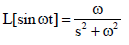QUESTION: 16

Laplace transform of (a + bt)2 where ‘a’ and ‘b’ are constants is given by:

Solution:

Ans.(c)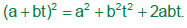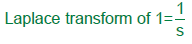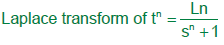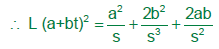QUESTION: 17

A delayed unit step function is defined as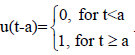Its Laplace transform is

Solution:

Ans. (d)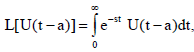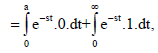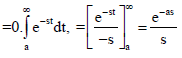QUESTION: 18

The Laplace transform of the function sin2 2t is

Solution:

Ans.(a)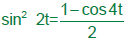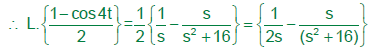QUESTION: 19

(s+1)-2 is the Laplace transform of

Solution:

Ans.(d)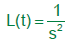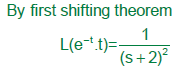QUESTION: 20

The running integrator, given by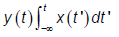Solution:

Ans. (b)

QUESTION: 21

The state transition matrix for the system  X- = AX with initial state X(0) is

Solution:

Ans. (c)

QUESTION: 22

The Fourier transform of x(t) = e–at u(–t), where u(t) is the unit step function

Solution:

Ans. (d)

QUESTION: 23

The fundamental period of the discrete-time signal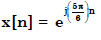is

Solution:

Ans. (b)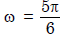or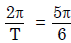or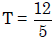QUESTION: 24

u(t) represents the unit step function. The Laplace transform of u(t – ζ) is

Solution:

Ans. (c)
f(t) = u(t – ζ)
L{f(t)} = L{u(t – ζ)}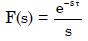QUESTION: 25

The fundamental period of x(t) = 2 sin πt + 3 sin 3πt, with t expressed in seconds, is

Solution:

Ans. (d)
H.C.F. of 2π and 3π is 6π.
Then, fundamental frequency = 6π
∴ Period, T =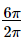= 3 sec

QUESTION: 26

If the Fourier transform of x[n] is X(e), then the Fourier transform of (–1)n x[n] is

Solution:

Ans. (c)

QUESTION: 27

Given f(t) and g(t) as shown below: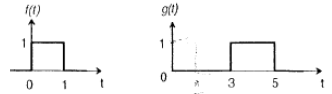g (t) can be expressed as

Solution:
QUESTION: 28

Given f(t) and g(t) as shown below:The Laplace transform of g(t) is

Solution:

Ans. (c)

QUESTION: 29

The Laplace transform of g(t) is

Solution:

Ans. (c)

QUESTION: 30

Let Y(s) be the Laplace transformation of the function y (t), then final value of the function is

Solution:

Ans. (c)